# Shortest Distance between a Point and a Circle

What is the distance between a circle $C$ with equation ${x}^{2}+{y}^{2}={r}^{2}$ which is centered at the origin and a point $P\left({x}_{1},{y}_{1}\right)$ ?

The ray $\stackrel{\to }{OP}$ , starting at the origin $O$ and passing through the point $P$ , intersects the circle at the point closest to $P$ . So, the distance between the circle and the point will be the difference of the distance of the point from the origin and the radius of the circle.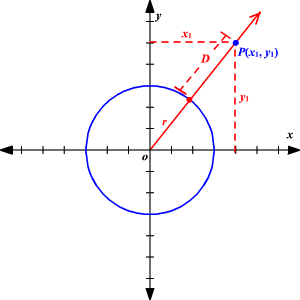Using the Distance Formula , the shortest distance between the point and the circle is $|\sqrt{{\left({x}_{1}\right)}^{2}+{\left({y}_{1}\right)}^{2}}-r\text{\hspace{0.17em}}|$ .

Note that the formula works whether $P$ is inside or outside the circle.

If the circle is not centered at the origin but has a center say $\left(h,k\right)$ and a radius $r$ , the shortest distance between the point $P\left({x}_{1},{y}_{1}\right)$ and the circle is $|\sqrt{{\left({x}_{1}-h\right)}^{2}+{\left({y}_{1}-k\right)}^{2}}-r\text{\hspace{0.17em}}|$ .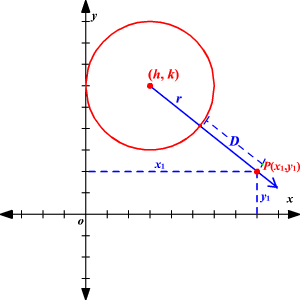Example 1:

What is the shortest distance between the circle ${x}^{2}+{y}^{2}=9$ and the point $A\left(3,4\right)$ ?

The circle is centered at the origin and has a radius $3$ .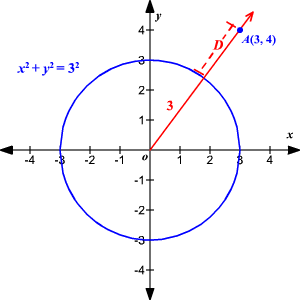So, the shortest distance $D$ between the point and the circle is given by

$\begin{array}{l}D=|\text{\hspace{0.17em}}\sqrt{{\left(3\right)}^{2}+{\left(4\right)}^{2}}-3\text{\hspace{0.17em}}|\\ \text{\hspace{0.17em}}\text{\hspace{0.17em}}\text{\hspace{0.17em}}\text{\hspace{0.17em}}\text{\hspace{0.17em}}=|\text{\hspace{0.17em}}\sqrt{25}-3\text{\hspace{0.17em}}|\\ \text{\hspace{0.17em}}\text{\hspace{0.17em}}\text{\hspace{0.17em}}\text{\hspace{0.17em}}\text{\hspace{0.17em}}=|\text{\hspace{0.17em}}5-3\text{\hspace{0.17em}}|\\ \text{\hspace{0.17em}}\text{\hspace{0.17em}}\text{\hspace{0.17em}}\text{\hspace{0.17em}}\text{\hspace{0.17em}}=2\end{array}$

That is, the shortest distance between them is $2$ units.

Example 2:

What is the shortest distance between the circle ${x}^{2}+{y}^{2}=36$ and the point $Q\left(-2,2\right)$ ?

The circle is centered at the origin and has a radius $6$ .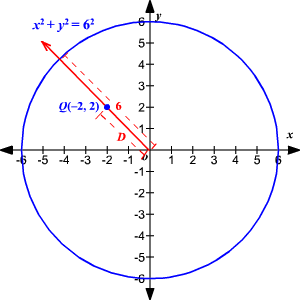So, the shortest distance $D$ between the point and the circle is given by

$\begin{array}{l}D=|\sqrt{{\text{\hspace{0.17em}}\left(-2\right)}^{2}+{\left(2\right)}^{2}}-6\text{\hspace{0.17em}}|\\ \text{\hspace{0.17em}}\text{\hspace{0.17em}}\text{\hspace{0.17em}}\text{\hspace{0.17em}}\text{\hspace{0.17em}}=|\text{\hspace{0.17em}}\sqrt{8}-6\text{\hspace{0.17em}}|\\ \text{\hspace{0.17em}}\text{\hspace{0.17em}}\text{\hspace{0.17em}}\text{\hspace{0.17em}}\text{\hspace{0.17em}}=6-2\sqrt{2}\\ \text{\hspace{0.17em}}\text{\hspace{0.17em}}\text{\hspace{0.17em}}\text{\hspace{0.17em}}\text{\hspace{0.17em}}\approx 3.17\end{array}$

That is, the shortest distance between them is about $3.17$ units.

Example 3:

What is the shortest distance between the circle ${\left(x+3\right)}^{2}+{\left(y-3\right)}^{2}={5}^{2}$ and the point $Z\left(-2,0\right)$ ?

Compare the given equation with the standard form of equation of the circle,

${\left(x-h\right)}^{2}+{\left(y-k\right)}^{2}={r}^{2}$ where $\left(h,k\right)$ is the center and $r$ is the radius.

The given circle has its center at $\left(-3,3\right)$ and has a radius of $5$ units.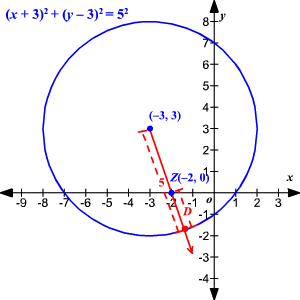Then, the shortest distance $D$ between the point and the circle is given by

$\begin{array}{l}D=|\text{\hspace{0.17em}}5-\sqrt{{\left(-3-\left(-2\right)\right)}^{2}+{\left(3-0\right)}^{2}}|\\ \text{\hspace{0.17em}}\text{\hspace{0.17em}}\text{\hspace{0.17em}}\text{\hspace{0.17em}}\text{\hspace{0.17em}}=|\text{\hspace{0.17em}}5-\sqrt{1+9}\text{\hspace{0.17em}}|\\ \text{\hspace{0.17em}}\text{\hspace{0.17em}}\text{\hspace{0.17em}}\text{\hspace{0.17em}}\text{\hspace{0.17em}}=|\text{\hspace{0.17em}}5-\sqrt{10}\text{\hspace{0.17em}}|\\ \text{\hspace{0.17em}}\text{\hspace{0.17em}}\text{\hspace{0.17em}}\text{\hspace{0.17em}}\text{\hspace{0.17em}}\approx 1.84\end{array}$

That is, the shortest distance between them is about $1.84$ units.

Example 4:

What is the shortest distance between the circle ${x}^{2}+{y}^{2}-8x+10y-8=0$ and the point $P\left(-4,-11\right)$ ?

Rewrite the equation of the circle in the form ${\left(x-h\right)}^{2}+{\left(y-k\right)}^{2}={r}^{2}$ where $\left(h,k\right)$ is the center and $r$ is the radius.

$\begin{array}{l}{x}^{2}+{y}^{2}-8x+10y-8=0\\ {x}^{2}-8x+16+{y}^{2}+10y+25=8+16+25\\ {\left(x-4\right)}^{2}+{\left(y+5\right)}^{2}=49\\ {\left(x-4\right)}^{2}+{\left(y+5\right)}^{2}={7}^{2}\end{array}$

So, the circle has its center at $\left(4,-5\right)$ and has a radius of $7$ units.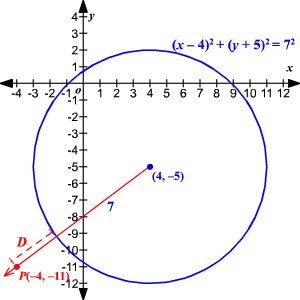Then, the shortest distance $D$ between the point and the circle is given by

$\begin{array}{c}D=|\text{\hspace{0.17em}}\sqrt{{\left(-4-4\right)}^{2}+{\left(-11-\left(-5\right)\right)}^{2}}-7\text{\hspace{0.17em}}|\\ =|\text{\hspace{0.17em}}\sqrt{64+36}-7\text{\hspace{0.17em}}|\\ =\sqrt{100}-7\\ =3\end{array}$

That is, the shortest distance between them is $3$ units.